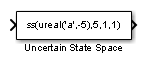Documentation

# Uncertain State Space

Specify uncertain system in Simulink

## Description

The Uncertain State Space block lets you model parametric and dynamic uncertainty in Simulink®. The block accepts uncertain state space (`uss)` models or any model that can be converted to `uss`, such as `umat`, `ureal` and `ultidyn` objects.## Parameters

### Uncertain system variable (uss)

Linear state-space model with uncertainty (`uss` object). Specify an `uss` object using one of the following:

• Function or expression that evaluates to an `uss` object. For example:

• `ss(ureal('a',-5),5,1,1)`

• `wt*input_unc`, where input_unc is an `ultidyn` object and `wt` and `input_unc` are defined in the MATLAB® workspace.

• Variable name, defined in the MATLAB workspace. For example, `unc_sys`, where you define `unc_sys` = `ss(ureal('a',-5),5,1,1) `in the workspace. This returns an `uss `object`.`

• Model type that can be converted to an `uss` object. For example:

• LTI models (`tf`, `zpk` and `ss`)

• Uncertain matrix (`umat`)

• Uncertain real parameters (`ureal`)

• Uncertain dynamics (`ultidyn`).

When the block is in a model with synchronous state control (see the State Control block), you must specify a discrete-time model.

### Uncertainty value (struct or [] to use nominal value)

Values of uncertain variables. The `uss` object that you enter in the Uncertain system variable (uss) field depends on uncertain variables (`ureal` or `ultidyn` object). Use this field to specify the values of these uncertain variables to use for simulation or linearization. Specify the value as one of the following:

Value

Description

`[]`

Use nominal values.

Structure

Use user-defined values. For example, `struct('a',1)` specifies a value of 1 for the uncertain variable `a`.

Use `ufind` and `usample` to generate randomized values of uncertain variables for Monte Carlo simulation. For more information, see Vary Uncertainty Values Using Individual Uncertain State Space Blocks and Vary Uncertainty Values Across Multiple Uncertain State Space Blocks in the Robust Control Toolbox User's Guide.

### Initial states (nominal dynamics)

If the nominal value of the uncertain state variable, `unc_sys.NominalValue where unc_sys` is the uncertain system variable specified in the Uncertain system variable field, has states, specify the initial condition for these states. The value defaults to zero.

### Initial states (uncertain dynamics)

If the uncertain system contains some dynamic uncertainty (`ultidyn`), specify the initial state of these dynamics. The value defaults to zero.